# Chapter V: Further Explorations of the Capital Asset Pricing Model

## An Introduction to Investment Theory

YALE School of Management

### Chapter V: Further Explorations of the Capital Asset Pricing Model

I. Risk-Return Tradeoff: A Technical Aside

Recall from last chapter that, when investors are well-diversified, they evaluate the attractiveness of a security based upon its contribution to portfolio risk, rather than its volatility per se. The intuition is that an asset with a low correlation to the tangency portfolio is desirable, because it shifts the frontier to the left.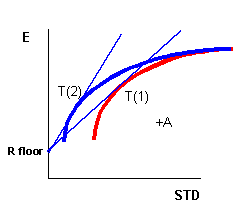This institution was formalized by Stephen Ross in an article called Finance, published in The New Palgrave. It is a simple argument that shows the theoretical basis for the "pricing" part of the Capital Asset Pricing Model.

Here goes: Suppose you are an investor who holds the market portfolio m and you are considering the purchase of a quantity dx of asset A, by financing it via borrowing at the riskless rate. This augments the return of the market portfolio by the quantity:

### dEm = [E A - Rf]dx

Where d symbolizes a small quantity change. This investment also augments the variance of the market portfolio. The variance of the market portfolio after adding the new asset is:

### v + dv = v + 2dx cov(A,m) + (dx)2 var(a)

The change in the variance is then:

### dv = 2 dx cov(A,m) + (dx)2 var(A)

For small dx's this is approximately:

### dv = 2 dx cov(A,m)

This gives us the risk-return tradeoff to investing in a small quantity of A:

### Risk-Return Tradeoff for A = dEm/dv = [E A - Rf]/ 2 cov(A,m)

Now, if the expected return of asset A is in equilibrium, then an investor should be indifferent between augmenting his or her portfolio with a quantity of A and simply levering up the existing market portfolio position. If this were NOT the case, then either the investor would not be willing to hold A, or A would dominate the portfolio entirely. We can calculate the same Risk-Return Tradeoff for buying dx quantity of the market portfolio P instead of security A.

### Risk-Return Tradeoff for P = dEm/dv = [E m - Rf]/ 2 var(m)

The equations are almost the same, except that the cov(A,m) is replaced with var(m). This is because the covariance of any security with itself is the variance of the security. These Risk-Reward Tradeoffs must be equal:

### Thus, [E A - Rf] = [cov(A,m)/var(m)][E m - Rf]

The value cov(A,m)/var(m) is also known as the ß of A with respect to m. ß is a famous statistic in finance. It is functionally related to the correlation and the covariance between the security and the market portfolio in the following way: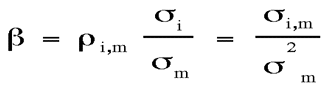II. A Model of Expected Returns

In the preceding example, notice that we used the expression expected returns. That is, we found an equation that related the expected future return of asset A (in excess of the riskless rate) to the expected future return of the market (in excess of the riskless rate). This expected return is the return that investors will demand when asset prices are in the equilibrium described by the CAPM. For any asset i, the CAPM argues that the appropriate rate at which to discount the cashflows of the firm is that same rate that investors demand to include the security in their portfolio:One surprising thing about this equation is what is not in it. There is no measure of the security's own standard deviation. The CAPM says that you do not care about the volatility of the security. You only care about its beta with respect to the market portfolio! Risk is now re-defined as the quantity of exposure the security has to fluctuations in the market portfolio.

III. The Security Market Line

The CAPM equation describes a linear relationship between risk and return. Risk, in this case, is measured by beta. We may plot this line in mean and ß space: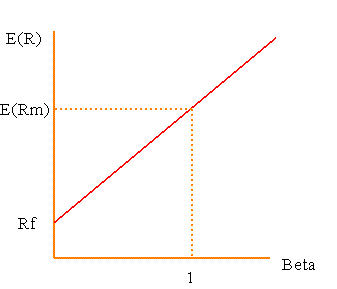One remarkable fact that comes from the linearity of this equation is that we can obtain the beta of a portfolio of assets by simply multiplying the betas of the assets by their portfolio weights. For instance the beta of a 50/50 portfolio of two assets, one with a beta of .8 and the other with a beta of 1 is .9. Easy!

The line also extends out infinitely to the right, implying that you can borrow infinite amounts to lever up your portfolio.

Why is the line straight? Well, suppose it curved, as the blue line does in the figure below. The figure shows what could happen. An investor could borrow at the riskless rate and invest in the market portfolio. Any investment of this type would provide a higher expected return than a security which lies on the curved line below. In other words, the investor could receive a higher expected return for the same level of systematic risk. In fact, if the security on the curve could be sold short, then the investor could take the proceeds from the short sale and enter into the levered market position -- generating an arbitrage in expectation.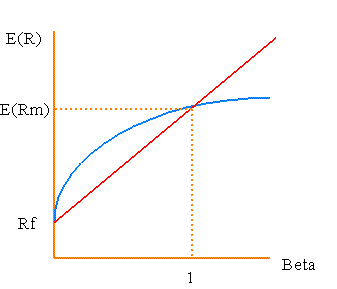IV. Expectations vs. Realizations

It is important to stress that the vertical dimension in the security market line picture is expected return. Things rarely turn out the way you expect. However, the CAPM equation also tells us about the realized rate of return. Since the realization is just the expectation plus random error, we can write:

### R i = Rf + ßi [ Rm - Rf ] + ei

This is useful, because it tells us that when we look at past returns, they will typically deviate from the security market line -- not because the CAPM is wrong, but because random error will push the returns off the line. Notice that the realized R m does not have to behave as expected, either. So, even the slope of the security market line will deviate from the average equity risk premium. Sometimes it will even be negative!

V. An Example

The appeal of the CAPM is clear -- it radically simplifies an inherently complex and troublesome problem. The question of the appropriate discount rate becomes virtually a back-of-the-envelope calculation! In fact, if you know a security's beta, estimating the discount rate is a snap: multiply beta times the expected risk premia of the market portfolio over the riskless rate.

For example, suppose you are a banker considering a private equity investment in a company with a new drug process. The process is inherently risky, i.e. the standard deviation of the project is 75% per year. The beta of the project is .5. The Rf = 5% and the E[Rm] = 13.5%. What is the required rate of return on the project?

Theory tells us that the answer does not depend upon the volatility associated with the returns. Instead we use the beta of the project.

### E[Rdrug]= 5% + (.5)(13.5% - 5%) = 9.25%

This is the required rate of return on the project. The answer would not change if the range of outcome next year broadened or narrowed. The ß is the only relevant piece information -- now all that remains is to estimate it!

VI. How Do You Estimate ß?

ß may be all we need, but it is not immediately clear how it should be estimated. What we really need is a quantitative estimate of how the future return changes in response to future changes in the world market portfolio. Good Luck! It is tough to even guess the empirical composition of the market portfolio, let alone estimate a beta. In practice (although it is not theoretically justified) analysts typically use the S&P 500 equity risk premium in this calculation. To estimate beta, regress the security returns for the past several periods (usually 60 months) on the market returns. The slope in this regression is an estimate of ß.Notice that this shows concretely that empirical property of ß as it measures the co-movement of the security with the market. Unfortunately, since the S&P 500 is not the world market portfolio, we are somewhat in the dark about how well this beta measures the true systematic risk.

VII. Assessing the CAPM

The CAPM is a classical model in finance. It is an equilibrium argument that, if true, answers most important investment questions. It tells us where to invest, how to invest and what discount rate to use for project cash flows. Not only that, it is a disarmingly simple model. The expected return of a security depends upon a simple statistic: ß. The relationship between risk and return is linear. Calculation of portfolio risk is trivial. At the same time, the CAPM is revolutionary. It tells us that the variance of a project is NOT a factor in determining the appropriate, risk-adjusted discount rate. It turns financial research from roll-up-your-sleeves fundamental analysis into a statistics problem. In short, the CAPM turned Wall Street on its head.

VIII. Conclusion. Is the CAPM True?

Here comes the bad news. Despite twenty years of attempts to verify or refute the Capital Asset Pricing Model, there is no consensus on its legitimacy. There are a few hints that the model is incorrect. For starters, we all hold different portfolios. Therefore, it cannot be exactly true. Researchers have focused upon the more interesting issue of whether rates of return depend upon ß and whether the elegant, linear form of the model holds for stocks. What they have found is that real markets typically deviate broadly from the exact model. While there are long periods in U.S. Capital market history when realized returns are positively related to betas, there are also long periods when they are not. Among the most forceful arguments against the CAPM advanced in recent times is a study by Eugene Fama and Kenneth French. These authors found that beta did a relatively poor job at explaining differences in the actual returns of portfolios of U.S. stocks. Instead, Fama and French noted that there were other variables besides beta with respect to the market that explained returns. Some of these were "fundamental" ratios long used by financial analysts in the pre-CAPM era such as Book to Market Ratio and Earnings Price Ratio. Another was simply the relative size of the company. The evidence against the CAPM continues to grow and despite its elegance, most researchers have turned to more more complex, but more powerful models.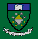© William N. Goetzmann